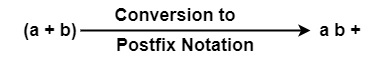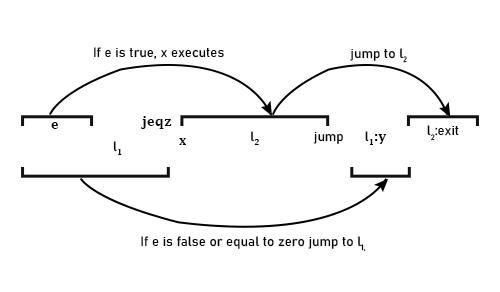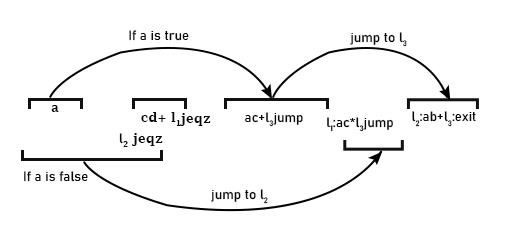# What is Postfix Notation?

In postfix notation, the operator appears after the operands, i.e., the operator between operands is taken out & is attached after operands.Example1 − Translate a ∗ d − (b + c) into Postfix form.

Solution

Example2 − Convert a + (b ∗⊝ c) is in Postfix form.

Solution

Here ⊝ represents the unary minus operator.

a b c ⊝ ∗ +

Example3 − Convert Postfix Expression into Infix Expression using Stack Implementation

a d ∗ bc + −

Solution

String SymbolsStack
AA
*(a * d)
B(a * d)b
C(a * d)b c
+(a ∗ d)(b + c)
-(a ∗ d)-(b + c)

Example4 − Calculate the value for the postfix expression.

57 + 2 ∗ 3/

Solution

This expression can be evaluated by using a stack. Each symbol can be inserted sequence wise & the operator can be applied on operands lying at the top of the stack and next to it.

SymbolStackDescription
55
75 75 + 7
+12
212 2
*2412 * 2
324 3
/824/3

Postfix Notations used in Control Statements

• Jump − Jump to label 𝑙 can be written in postfix notations as −

𝑙jump

• jlt (Jump if less than) − e1 e2 𝑙 jlt makes the jump to label if e1 has a smaller value than e2.

• jeqz (jump if equal to zero) − e 𝑙 jeqz causes jump to 𝑙 if e has the value zero.

Example5 − Convert the following statement into postfix Notation.

if e then x else y

Solution

If − else statement can be written as

If e then

x

else

𝑙1: y

𝑙2: exit

The postfix Notation will beExample6 − Convert expression into Postfix Notation.

if a then if c + d then a + c else a * c else a + b.

Solution

It can be written as

if a then

if c + d then

a + c

else

a * c

𝑙1:

else

𝑙2: a + b

𝑙3: exit